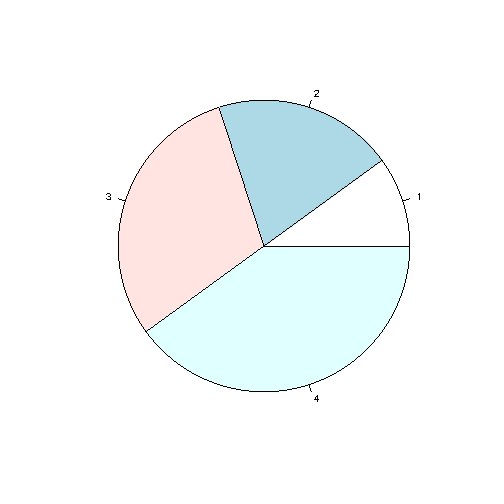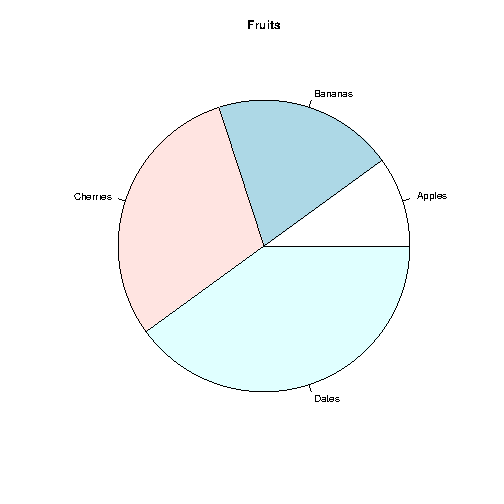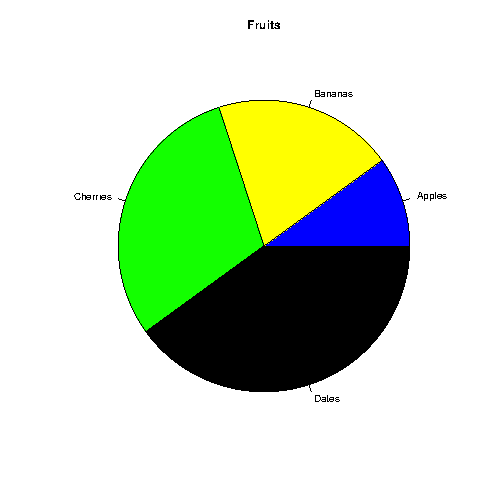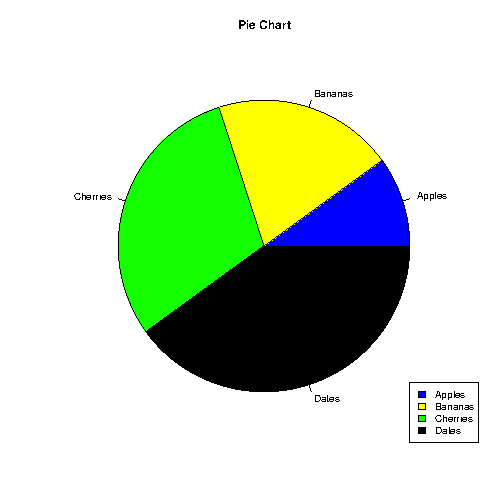# R Pie Charts

## Pie Charts

A pie chart is a circular graphical view of data.

Use the `pie()` function to draw pie charts:

### Example

# Create a vector of pies
x <- c(10,20,30,40)

# Display the pie chart
pie(x)

Result:Try it Yourself »

#### Example Explained

As you can see the pie chart draws one pie for each value in the vector (in this case 10, 20, 30, 40).

By default, the plotting of the first pie starts from the x-axis and move counterclockwise.

Note: The size of each pie is determined by comparing the value with all the other values, by using this formula:

The value divided by the sum of all values: `x/sum(x)`

## Start Angle

You can change the start angle of the pie chart with the `init.angle` parameter.

The value of `init.angle` is defined with angle in degrees, where default angle is 0.

### Example

Start the first pie at 90 degrees:

# Create a vector of pies
x <- c(10,20,30,40)

# Display the pie chart and start the first pie at 90 degrees
pie(x, init.angle = 90)

Result:Try it Yourself »

Use the `label` parameter to add a label to the pie chart, and use the `main` parameter to add a header:

### Example

# Create a vector of pies
x <- c(10,20,30,40)

# Create a vector of labels
mylabel <- c("Apples", "Bananas", "Cherries", "Dates")

# Display the pie chart with labels
pie(x, label = mylabel, main = "Fruits")

Result:Try it Yourself »

## Colors

You can add a color to each pie with the `col` parameter:

### Example

# Create a vector of colors
colors <- c("blue", "yellow", "green", "black")

# Display the pie chart with colors
pie(x, label = mylabel, main = "Fruits", col = colors)

Result:Try it Yourself »

## Legend

To add a list of explanation for each pie, use the `legend()` function:

### Example

# Create a vector of labels
mylabel <- c("Apples", "Bananas", "Cherries", "Dates")

# Create a vector of colors
colors <- c("blue", "yellow", "green", "black")

# Display the pie chart with colors
pie(x, label = mylabel, main = "Pie Chart", col = colors)

# Display the explanation box
legend("bottomright", mylabel, fill = colors)

Result:Try it Yourself »

The legend can be positioned as either:

`bottomright`, `bottom`, `bottomleft`, `left`, `topleft`, `top`, `topright`, `right`, `center`

W3Schools is optimized for learning and training. Examples might be simplified to improve reading and learning. Tutorials, references, and examples are constantly reviewed to avoid errors, but we cannot warrant full correctness of all content. While using W3Schools, you agree to have read and accepted our terms of use, cookie and privacy policy.